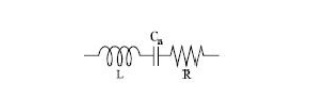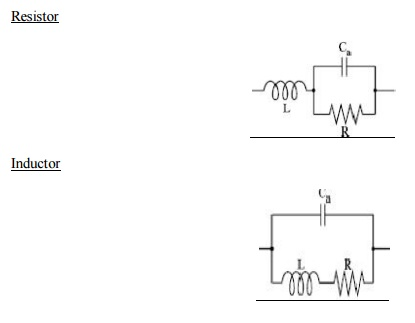Home | | RF and Microwave Engineering | Important Questions and Answers: Two Port RF Networks - Circuit Representation

# Important Questions and Answers: Two Port RF Networks - Circuit Representation

RF and Microwave Engineering - Two Port RF Networks - Circuit Representation - Important Questions and Answers: Two Port RF Networks - Circuit Representation

TWO PORT RF NETWORKS –CIRCUIT REPRESENTATION

1.Define Microwave?

Microwaves are electromagnetic waves with wavelengths ranging from 1 mm to 1 m, or frequencies between 300 MHz and 300 GHz.

2.Define Scattering matrix?

Scattering matrix is a squre matrix which gives all the combination of power relationship between the

various input and output ports.

3.Mention the limitation in measuring Z,Y and ABCD parameters at microwave frequencies

ü Non availability of terminal voltage and current measuring equipment

ü Short circuit and open circuit are nit easily achieved for a wide range of frequencies

ü Presence of active devices makes the circuit unstable for short or open circuit

4.A 5dB attenuator is specified as having VSWR of 1.2 assuming that the device is reciprocal find S parameters.

Attenuation dB for any devices is = -20log [s12]

Log s12 = -5/20 = -0.25

(or) [s12] = 10 -0.25 = 0.56

Thus  Г = s11 =VSWR -1/VSWR+1

=       1.2 – 1/1.2+1  = 0.2/2.22 = 0.091

4. Name the properties of S – Parameters?

It possess symmetric property sij =sji

It possess unitary property [s][s]*=[i]

6.Draw the equivalent circuit for practical capacitor7.Define reciprocal and symmetrical networks?

The reciprocal device has a same transmission characteristics in either direction of a pair of ports and is characterized by a symmetric scattering matrix

Sij = Sji  ;  i !=j

Which results [s]t = [s]

8.Express power input and power output under matched conditions for a two port network in terms of wave components

Pin = ½ [an]2

Prn = ½ [bn]2

9. Draw the equivalent circuit for a inductor at radio frequency10.List any four reasons for wide use of RF?

ü Because of high operating frequency microwave system can carry large quantities of information

ü High frequency mean short wavelength , which require relatively small antennas

ü Microwave signals are more easily propagated around physical obstacles , such as water and high mountains

ü Fewer repeaters are necessary for amplification

ü Increased bandwidth availability

ü Minimal cross talk

11.Give the relationship between [s] and [z] matrix13. Draw the high frequency equivalent circuit for resistor and inductor

Resistor14. Why the s-parameters are used in microwaves?

The H, Y, Z and ABCD parameters are difficult at microwave frequencies due to the following reasons. (i)Equipment is not readily available to measure total voltage and total current at the ports of the network.

(ii)             Short circuit and open circuit are difficult to achieve over a wide range of frequencies.

(iii)           Presence of active devices makes the circuit unstable for short or open circuit.

(iv)           Therefore microwave circuits are analyzed using scattering or S parameters which linearly relate the reflected wave amplitude with those of incident waves.

15 .What is the Scattering matrix for N port device?

[S]= S11 S12 S13…… S1n S21 S22 …………S2n

S31 S32 ………….S3n

. .

. .

Sm1 Sm2 ………...Smm

16.            Give the application of microwave

ü Satellite communication

ü Telemetry

ü Microwave oven

ü Transmission of video signals

ü Microwave diathermy

Study Material, Lecturing Notes, Assignment, Reference, Wiki description explanation, brief detail
RF and Microwave Engineering : Two Port RF Networks : Circuit Representation : Important Questions and Answers: Two Port RF Networks - Circuit Representation |